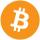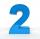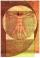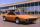# Pupils

There are 32 pupils in the classroom, and girls are two-thirds more than boys.

a) How many percents are more girls than boys? Round the result to a whole percentage.
b) How many are boys in the class?
c) Find the ratio of boys and girls in the class. Write in the simplest form.

Result

c =  12
d =  20
p =  67 %
f =  3:5

#### Solution:

c+d=32
d = c+ 2/3c

c+d = 32
5c-3d = 0

c = 12
d = 20

Calculated by our linear equations calculator.Leave us a comment of example and its solution (i.e. if it is still somewhat unclear...):

Showing 0 comments:Be the first to comment!#### To solve this verbal math problem are needed these knowledge from mathematics:

Need help calculate sum, simplify or multiply fractions? Try our fraction calculator. Our percentage calculator will help you quickly calculate various typical tasks with percentages. Do you have a system of equations and looking for calculator system of linear equations?

## Next similar examples:

1. Three workshopsThere are 2743 people working in three workshops. In the second workshop works 140 people more than in the first and in third works 4.2 times more than the second one. How many people work in each workshop?
2. Holidays - on poolChildren's tickets to the swimming pool stands x € for an adult is € 2 more expensive. There was m children in the swimming pool and adults three times less. How many euros make treasurer for pool entry?
3. ParkingAt a building parking, 245 spaces are for cars, 56 are for vans and the rest are for buses. If 14% of all parking spaces are for buses, how many parking spaces are there in the building? How many spaces are for buses?
4. Flowers 2Cha cruz has a garden. The ratio roses to tulips is 2 : 5, the ratio of roses to orchids is 7 : 6. Cha cruz wonders what the ratio of tulips to orchids is. If Cha cruz has 183 plants, how many of each kind are there?
5. Boys to girlsThe ratio of boys to girls in a party is 3:5 . If 6 more boys arrived and 4 girls left the party, the ratio of boys to girls would be 5:6 . How many are in the party originally?
6. RewardThree workers have shared a common reward 13110 CZK follows: first worker got 35% less than the second and third worker got 20% more than the second worker. How much got each worker?
7. LegsCancer has 5 pairs of legs. The insect has 6 legs. 60 animals have a total of 500 legs. How much more are cancers than insects?
8. Profit gainIf 5% more is gained by selling an article for Rs. 350 than by selling it for Rs. 340, the cost of the article is:
9. Unknown amount of moneyDamian and Denis split an unknown amount in the ratio of 5:4 . Damian got six euros more than Denis. Calculate an unknown amount. Determine how much money got Damian and how Denis.
10. Elimination methodSolve system of linear equations by elimination method: 5/2x + 3/5y= 4/15 1/2x + 2/5y= 2/15
11. Three unknownsSolve the system of linear equations with three unknowns: A + B + C = 14 B - A - C = 4 2A - B + C = 0
12. Numbers at ratioThe two numbers are in a ratio 3:2. If we each increase by 5 would be at a ratio of 4:3. What is the sum of original numbers?
13. Percentage 1052 shorts and missed 13. Calculate percentage
14. Theorem proveWe want to prove the sentence: If the natural number n is divisible by six, then n is divisible by three. From what assumption we started?
15. Linsys2Solve two equations with two unknowns: 400x+120y=147.2 350x+200y=144
16. Golden ratioDivide line of length 14 cm into two sections that the ratio of shorter to greater is same as ratio of greater section to whole length of the line.
17. SpeedometerThe speedometer of a car reads 66 km/h. It's accurate to within 4.1% What is the maximum possible error?